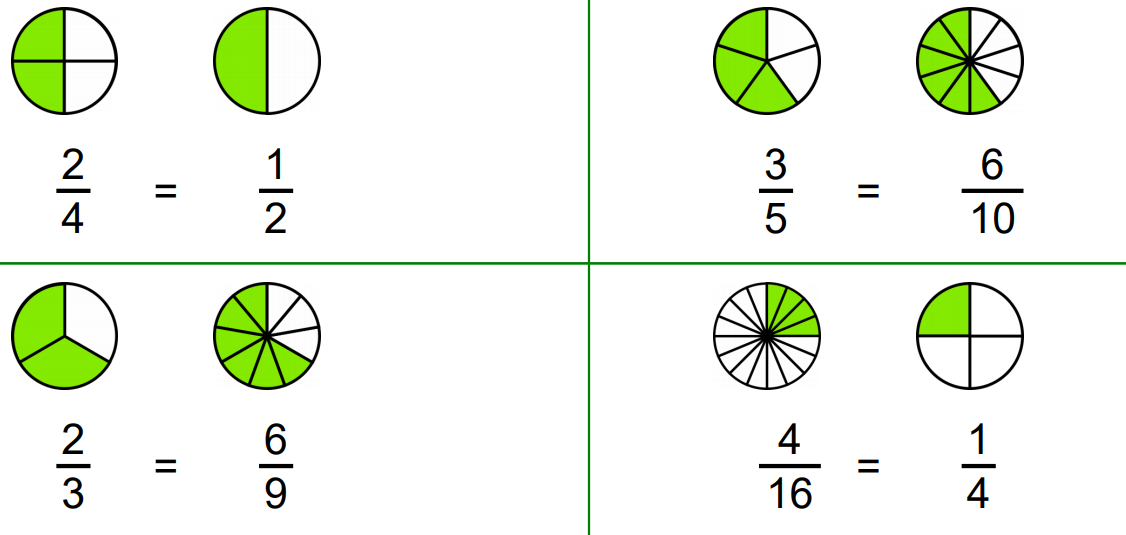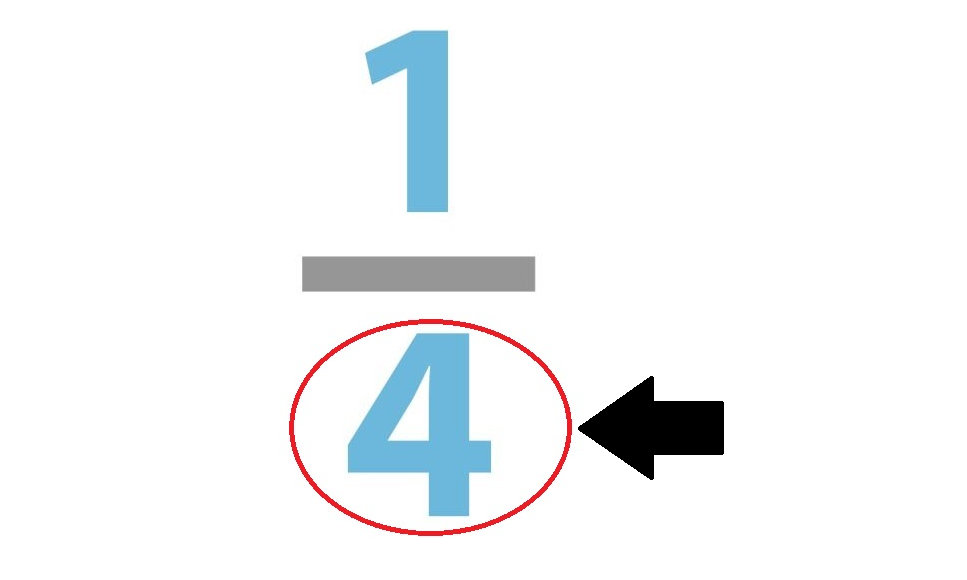# Start to Learn Fractions in Maths Today!

•## Chapters

Confused about the difference between a fraction, decimal, and a percentage? All of them are similar and serve the same function - to tell us how many parts of a collection are taken.

For example, imagine a pizza cut into five slices and your friend has already eaten three of them. If you want to understand how much of the collection(i.e. pizza) is taken you need to understand either fractions, decimals, or percentages.

In this article, we’ll teach you about fractions.## What is a fraction? 🤔

A fraction is a way to split up a whole number into equal parts. The two parts are the numerator and the denominator.

The top part of a fraction is called the numerator and the part of the fraction at the bottom is called the denominator.

For example, in the fraction 512,  5 is the numerator and 12 is the denominator.

Here’s another real-life example: Using any countable item (like forks or napkins) in your house count 8 pieces. This is the denominator. It is the number we divide our whole number into.

Now take one piece away and notice that this is 1 piece of 8 and therefore we would write the one as the numerator. This can be done with any number and can also be used to understand halves, quarters and eighths.

We like this method because it shows you that the denominator is often concrete. This is especially important when learning how to add and subtract fractions.

## Equivalent Fractions

When working with fractions, we often see two fractions and ask ourselves, “Aren’t these two the same thing?”

You’re probably right because we get things such as equivalent fractions. They are fractions that often have different denominators and numerators but, in the end, can be simplified to the same fraction.Look at the fractions above, all these fractions take up the same part of one whole.

These fractions, and recognising them, is important to the addition, subtraction, multiplication, and division of fractions.

So, keep this in mind as we move forward to the more difficult parts of fractions.

## Simplifying Fractions

This step actually comes at the very end of any of the sums we will teach you how to do, however it is very important that you know how to do this before we move on to the more difficult parts.

Simplification can easily be explained by considering how much space 12 boxes will take when we can fit everything that is in those twelve boxes into 2 📦. So why would we still use the 12 boxes? Why not recycle them or give them to someone who has enough things to fill all 12 boxes?

It is quite easy to do if you follow the method of just using your times tables to determine how much a fraction can be simplified.

So, if the denominator and the numerator can be divided by the same number, we do a simple division and we get the simplified version until we cannot divide the numbers any longer.

Not being able to divide further, usually happens when any of the two numbers become a prime number, or when the numbers obviously do not divide into each other (for example 3/4).

## The Addition ➕ and Subtraction ➖ of Fractions

There are two types of addition and subtraction of fractions found on this basic level. Adding and subtracting with the same denominator and with different denominators.

Just to recap, this means that when we subtract or add in fractions, our method depends on the denominator.If the denominator is the same, we use a simple method of subtracting or adding the numerators in each fraction from each other.

This is the method that most schools use all around the world. This method is tried and true, however you can take this method and apply it to something other than a pen and paper.

You can also try it out with objects around the house, or even a pizza! Alternatively, you could look at other methods to spice things up and make addition and subtraction easy.

Now we move on to the more difficult method of adding fractions that have different denominators.

How do we do this? We change the denominator before we complete any other subtraction or addition.

Let's say we have two denominators, 3 and 7, and each of those fractions have different numerators. We take the two denominators and change them to represent the same number. The easiest way to do this is by multiplying the 3 and 7, giving us 21.

However remember this step – Whatever you do to the denominator of each fraction, you must do with the numerator.

The last step to any fraction equation, is simplifying the final fraction. Sometimes, however, this can’t be done so there is no need to worry about it.

Keep in mind that both methods can be used for subtraction and addition. But often, the longer a method is, the easier it is for us to have trouble with it or miss a step.

## Multiplying fractions ✖️

To multiply a fraction is as simple as multiplying the numerators and then multiplying the denominators. However, we can’t leave the fraction this way!

We go ahead and simplify it, and then we have our final answer.

The easiest way to learn this concept is by taking the fraction apart. Move both numerators to one line and multiply. Then in the next line multiply only the denominators. Then just draw a line between the two answers to symbolise a fraction. The simplification can come later. It's better and, in the end, easier to learn one concept at a time when it comes to learning something as difficult as multiplying and dividing fractions.

So, unless you have understood simplification well, do not push yourself.

## Dividing Fractions ➗

To learn dividing fractions just follow this  3-step method.

Step 1: Leave the first fraction alone 🙅‍♀️

Step 2: Change the divide to a multiply ➗➡️✖️

Step 3: Invert the Numerator and the Denominator in the final fraction ↕️

That’s it! Follow these 3 easy steps, simplify (of course) and there you can divide any fraction that you need to.

## Other tips and rules 👍

Here are some other alternative tips when it comes to learning fractions:

Tip 1: Try dividing up cups into other fractions. This is a method to learn fractions of a whole number.

Tip 2: Make fraction facts about food, patches of flowers in your garden or places in your home. State, in fractions, how many rooms have windows, how much of the pizza has all the toppings on, or how much each patch of flowers takes up of the garden. This method also teaches you estimation, another key component to fraction calculations.

Tip 3: Try using online visuals, with fun maths games and images all over the internet.

Tip 4: Do not be afraid to use calculators sometimes.

It's important to remember to connect fractions to decimals or whole numbers. Never forget that when a numerator is a number place higher than the denominator, that means you have a whole number to include.

## Still struggling?

There is no shame in not being able to connect these ideas or connect to maths! Fractions are difficult, as are a lot of other things in maths, but have faith and keep trying.

If you are still struggling, don’t hesitate to book a trial lesson with one of our GoStudent maths tutors!  They will be able to ground all of these methods, and help you with creative ways to learn maths.